# JP6581017B2 - Rotating electric machine - Google Patents

## Info

Publication number
JP6581017B2
JP6581017B2 JP2016036153A JP2016036153A JP6581017B2 JP 6581017 B2 JP6581017 B2 JP 6581017B2 JP 2016036153 A JP2016036153 A JP 2016036153A JP 2016036153 A JP2016036153 A JP 2016036153A JP 6581017 B2 JP6581017 B2 JP 6581017B2
Authority
JP
Japan
Prior art keywords
degrees
damper
fixed support
mass
opening angle
Prior art date
Legal status (The legal status is an assumption and is not a legal conclusion. Google has not performed a legal analysis and makes no representation as to the accuracy of the status listed.)
Active
Application number
JP2016036153A
Other languages
Japanese (ja)
Other versions
JP2017153335A (en
Inventor

Original Assignee

Priority date (The priority date is an assumption and is not a legal conclusion. Google has not performed a legal analysis and makes no representation as to the accuracy of the date listed.)
Filing date
Publication date
Application filed by 東芝三菱電機産業システム株式会社, 国立大学法人 長崎大学, 国立大学法人 長崎大学 filed Critical 東芝三菱電機産業システム株式会社
Priority to JP2016036153A priority Critical patent/JP6581017B2/en
Publication of JP2017153335A publication Critical patent/JP2017153335A/en
Application granted granted Critical
Publication of JP6581017B2 publication Critical patent/JP6581017B2/en
Active legal-status Critical Current
Anticipated expiration legal-status Critical

• 230000000694 effects Effects 0.000 description 11
• 238000006073 displacement reaction Methods 0.000 description 9
• 230000001629 suppression Effects 0.000 description 8
• 238000004458 analytical method Methods 0.000 description 6
• 238000000034 method Methods 0.000 description 5
• 210000001015 Abdomen Anatomy 0.000 description 4
• 239000006096 absorbing agent Substances 0.000 description 3
• 230000001133 acceleration Effects 0.000 description 2
• 230000000875 corresponding Effects 0.000 description 2
• 230000001419 dependent Effects 0.000 description 2
• 238000001816 cooling Methods 0.000 description 1
• 238000010586 diagram Methods 0.000 description 1
• 230000005489 elastic deformation Effects 0.000 description 1
• 239000008187 granular material Substances 0.000 description 1
• 230000004048 modification Effects 0.000 description 1
• 238000006011 modification reaction Methods 0.000 description 1
• 239000002245 particle Substances 0.000 description 1
• 239000000843 powder Substances 0.000 description 1

## Images

•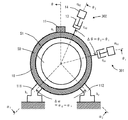•••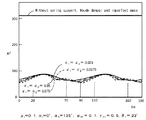•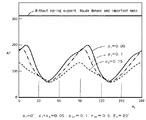•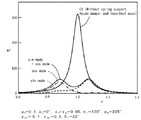•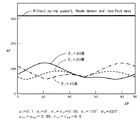••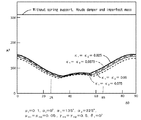•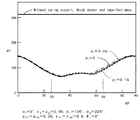•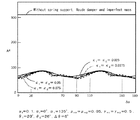••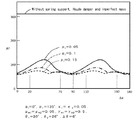•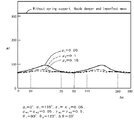•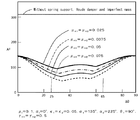••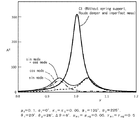•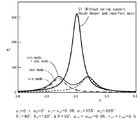## Description

The present invention relates to a rotating electrical machine including a non-uniform mass, a hood damper, and a plurality of support legs.
As a typical structure of a rotating electrical machine including an electric motor and a generator, a fixed support member including a cylindrical stator fixedly supported, and a rotor supported rotatably in the fixed support member Is widely known. In a rotating electrical machine, resonance occurs when the electromagnetic force frequency acting between the fixed support member and the rotor matches the natural frequency of the fixed support member, and the fixed support member vibrates to generate electromagnetic noise. There are things to do.
As a countermeasure for suppressing such vibration of the rotating electrical machine, particularly vibration in the annular vibration mode, a technique of attaching a dynamic vibration absorber to the rotating electrical machine is known (Patent Document 1).
In addition, a technique for suppressing vibration in consideration of the position of the support leg of the rotating electrical machine is known (Patent Document 2).
Moreover, the technique which suppresses the vibration of a motor with the damping member using a granular material is known (patent document 3).
Furthermore, a technique for changing the natural frequency by attaching a weight (non-uniform mass body) to the outside of the stator frame in order to suppress resonance at a specific rotational speed is also known (Patent Document 4).
JP 2014-57406 A JP 2013-150383 A JP 2000-46103 A JP 7-154940 A
In the said patent document 1, it is necessary to attach a dynamic vibration absorber, and it is not considered about the support leg of a rotary electric machine. In Patent Document 2, although consideration is given to the support leg of the rotating electrical machine, the support leg is handled as a mere fixed support and is not considered as an elastic support element.
Further, Patent Document 3 does not disclose anything about which position on the outer periphery of the motor is effective when the damping member using the powder particles is attached.
Furthermore, Patent Document 4 discloses a technique for changing the natural frequency by attaching a weight to the outside of the stator frame in order to suppress resonance at a specific rotational speed, but the relationship with the support legs and the like is disclosed. However, there is no disclosure about where to place the weight.
The present invention has been made in view of the above circumstances, and in a rotating electrical machine having a plurality of support legs, a dynamic damper is not used, a hood damper and a non-uniform mass body are used, and the support legs are elastically deformed. The purpose is to suppress vibration in consideration.
In order to achieve the above object, one aspect of a rotating electrical machine according to the present invention includes a cylindrical fixed support member, a rotor that is rotatably supported in the fixed support member, and a periphery of the fixed support member. and at least one heterogeneous mass is fixed in the direction of the predetermined position, the fixing and supporting the circumferential direction are arranged with a predetermined opening angle first and second support for supporting the fixed support member of the member And at least one leg that is spaced apart in the circumferential direction of the fixed support member relative to the non-uniform mass and is attached to the fixed support member to produce a resistance force against radial movement of the fixed support member A rotary electric machine having a hood damper, and taking an angular coordinate in a rotation direction of the rotor with a circumferential position of one of the at least one non-uniform mass body as an origin, Said first support The angular coordinate position and alpha 1, the angular coordinate position of the second support leg and alpha 2, and Δα = α 21, when the angular coordinate position of the hood damper as θ 1, α 1 is 30 to 60 degrees, 120 to 150 degrees, 210 to 240 degrees, 300 to 330 degrees, and Δα is 0 to 20 degrees, 70 to 110 degrees, 160 to 200 degrees, 250 to 290 degrees, It is any one of 340 to 360 degrees, and θ 1 is any one of 15 to 35 degrees, 105 to 125 degrees, 195 to 215 degrees, and 285 to 305 degrees.
Another aspect of the rotating electrical machine according to the present invention includes a cylindrical fixed support member, a rotor that is rotatably supported in the fixed support member, and a fixed position in a circumferential direction of the fixed support member. At least one of the heterogeneous mass is, the first and second support legs, wherein arranged at a predetermined opening angle in the circumferential direction of the fixed support member for supporting the fixed support member, said non-uniform A first hood damper and a second hood damper which are arranged apart from the mass body in the circumferential direction of the fixed support member and are attached to the fixed support member to generate a resistance force against a radial movement of the fixed support member; The rotary electric machine has the first position of the first non-uniform mass body as an origin and the angular coordinate in the rotational direction of the rotor as the origin. the angular coordinate position of the support legs α of And, said second angular coordinate position of the support legs and alpha 2, the angular coordinate position of the first hood damper and theta 1, the angular coordinates of the second hood damper and theta 2, [Delta] [alpha] = alpha 2− α 1 , Δθ = θ 2 −θ 1 , α 1 is 30 to 60 degrees, 120 to 150 degrees, 210 to 240 degrees, 300 to 330 degrees, and Δα is 0 -20 °, 70-110 °, 160-200 °, 250-290 °, 340-360 °, and θ 1 is 40-90 °, 130-180 °, 220-270 °, 310 ˜360 degrees, and Δθ is any of 25 to 65 degrees, 115 to 155 degrees, 205 to 245 degrees, and 295 to 335 degrees.
Another aspect of the rotating electrical machine according to the present invention includes a cylindrical fixed support member, a rotor that is rotatably supported in the fixed support member, and a fixed position in a circumferential direction of the fixed support member. At least one of the heterogeneous mass is, the first and second support legs, wherein arranged at a predetermined opening angle in the circumferential direction of the fixed support member for supporting the fixed support member, said non-uniform A first hood damper and a second hood damper which are arranged apart from the mass body in the circumferential direction of the fixed support member and are attached to the fixed support member to generate a resistance force against a radial movement of the fixed support member; The rotary electric machine has the first position of the first non-uniform mass body as an origin and the angular coordinate in the rotational direction of the rotor as the origin. the angular coordinate position of the support legs α of And, said second angular coordinate position of the support legs and alpha 2, the angular coordinate position of the first hood damper and theta 1, the angular coordinates of the second hood damper and theta 2, [Delta] [alpha] = alpha 2− α 1 , Δθ = θ 2 −θ 1 , α 1 is 30 to 60 degrees, 120 to 150 degrees, 210 to 240 degrees, 300 to 330 degrees, and Δα is 0 -20 °, 70-110 °, 160-200 °, 250-290 °, 340-360 °, and θ 1 is 10-30 °, 100-120 °, 190-210 °, 280 It is any one of -300 degree | times.
According to the present invention, in a rotating electrical machine having a plurality of support legs, it is possible to suppress vibration in consideration of elastic deformation of the support legs using a hood damper and a non-uniform mass body without using a dynamic vibration absorber. it can.
It is typical sectional drawing perpendicular | vertical to the rotating shaft of the rotary electric machine which concerns on embodiment of this invention. It is a figure which shows the example of distribution of the circumferential direction in sectional drawing perpendicular | vertical to the axis | shaft of a rotary electric machine of the electromagnetic force concerning the fixing support member of a rotary electric machine. In the rotating electrical machine according to the embodiment of the present invention, the hood damper position θ 1 and the dimensionless spring in the case of including one non-uniform mass body, two spring supports, and one hood damper (one damper case) It is a graph which shows the influence which constant (kappa) exerts on an amplitude. It is a graph which shows the influence which spring support opening angle (DELTA) (alpha) and dimensionless spring constant (kappa) have on an amplitude in the rotary electric machine (case with one damper) which concerns on embodiment of this invention. It is a graph which shows the influence which 1st spring support position (alpha) 1 and mass ratio (micro | micron | mu) I of a nonuniform mass body have on an amplitude in the rotary electric machine (case with one damper) which concerns on embodiment of this invention. 4 is a graph showing an example of a resonance curve in which the dimensionless frequency is taken on the horizontal axis and the dimensionless amplitude is taken on the vertical axis in the rotating electrical machine (case with one damper) according to the embodiment of the present invention. In the rotating electrical machine according to the embodiment of the present invention, when the two spring supports and the two hood dampers are provided (two damper cases), the damper opening angle Δθ and the first hood damper position θ 1 have an amplitude. It is a graph which shows the influence which it has, Comprising: It is a graph shown about the case where the influence of damper opening angle (DELTA) (theta) is small (1st group). An electric motor graph showing the damper opening angle Δθ in (damper two cases) and the 1 first hood damper position θ is the effect on the amplitude according to the embodiment of the present invention, the effect of the damper opening angle Δθ It is a graph shown about the case where it is large (2nd group). It is a graph which shows the influence which damper opening angle (DELTA) (theta) and dimensionless spring constant (kappa) exert on an amplitude in the 2nd group of the rotary electric machine (case with two dampers) which concerns on embodiment of this invention. It is a graph which shows the influence which damper opening angle (DELTA) (theta) and mass ratio (micro | micron | mu) I of a nonuniform mass body have on an amplitude in the 2nd group of the rotary electric machine (case with two dampers) which concerns on embodiment of this invention. It is a graph which shows the influence which the spring support opening angle (DELTA) (alpha) and dimensionless spring constant (kappa) have in an amplitude in the 1st group of the rotary electric machine (case with two dampers) which concerns on embodiment of this invention. It is a graph which shows the influence which the spring support opening angle (DELTA) (alpha) and the dimensionless spring constant (kappa) have on an amplitude in the 2nd group of the rotary electric machine (case with two dampers) which concerns on embodiment of this invention. It is a graph which shows the influence which the spring support opening angle | corner (DELTA) (alpha) and the mass ratio (micro | micron | mu) I of a nonuniform mass body have on an amplitude in the 1st group of the rotary electric machine (case with two dampers) concerning embodiment of this invention. It is a graph which shows the influence which the spring support opening angle (DELTA) (alpha) and the mass ratio (micro | micron | mu) I of a nonuniform mass body have on an amplitude in the 2nd group of the rotary electric machine (case with two dampers) concerning embodiment of this invention. It is a graph which shows the influence which damper opening angle | corner (DELTA) (theta) in the 2nd group of the rotary electric machine (case with two dampers) concerning this embodiment of the invention and mass ratio (micro | micron | mu) H1 = microH2 of a hood damper has on an amplitude. It is a graph which shows the influence which damper opening angle (DELTA) (theta) and the hood damper damping ratio (gamma) H1 = (gamma) H2 have in an amplitude in the 2nd group of the rotary electric machine (case with two dampers) which concerns on embodiment of this invention. 5 is a graph showing an example of a resonance curve in which a dimensionless frequency is taken on the horizontal axis and a dimensionless amplitude is taken on the vertical axis in the first group of the rotating electrical machine (case with two dampers) according to the embodiment of the present invention. 6 is a graph showing an example of a resonance curve in which a dimensionless frequency is taken on the horizontal axis and a dimensionless amplitude is taken on the vertical axis in the second group of the rotating electrical machine (case with two dampers) according to the embodiment of the present invention.
Hereinafter, an embodiment of a rotating electrical machine according to the present invention will be described with reference to the drawings.
First, an analysis method related to vibration suppression of an embodiment of a rotating electrical machine according to the present invention will be described.
FIG. 1 is a schematic cross-sectional view perpendicular to the rotation axis of the rotating electrical machine according to the embodiment of the present invention. FIG. 2 is a diagram showing an example of the distribution in the circumferential direction in a cross-sectional view perpendicular to the axis of the rotating electrical machine, of the electromagnetic force applied to the fixed support member of the rotating electrical machine.
In a hammering test, it is known that a mode having nodes in the axial direction cannot be obtained in a frequency range of several thousand Hz or less where electromagnetic vibration is a problem. Therefore, for the sake of simplicity, the stationary support member 10 including the stator of the rotating electrical machine and the outer stator frame is approximated by a uniform ring as shown in FIG. 1 that does not consider the axial distribution of displacement. I decided to. Here, the name of the “fixed support member” is “fixed” in the sense that the rotor 50 is supported without rotating, and when considering the vibration of the fixed support member 10, It is not fixed and vibrates.
It is assumed that the fixed support member 10 is cylindrical and has a uniform thickness in the circumferential direction. Inside the fixed support member 10, a rotor 50 that rotates around an axis common to the axis of the fixed support member 10 is disposed. A gap 51 is formed between the fixed support member 10 and the rotor 50.
A non-uniform mass body 11 is fixed to the outside of the fixed support member 10. There are P non-uniform mass bodies 11, and the angular position in the circumferential direction is α p (p = 1,..., P). However, here, it is assumed that P = 1, the position (α I ) of the one non-uniform mass body 11 is the origin, and the angular coordinate is taken in the rotational direction of the rotor 50 in the circumferential direction of the fixed support member 10. To do.
N spring supports (support legs) 111 and 112 are installed on the outside of the fixed support member 10 at a position of a circumferential angle θ = α n (n = 1,..., N). FIG. 1 shows a case where N = 2.
It is assumed that the fixed support member 10 performs ring vibration. Through the spring support 111 and 112 of the spring constant k n the stationary support member 10 and radially intended to elastically supported relative to a fixed position.
Furthermore, J food dampers (Hood Dampers) 301 and 302 are installed outside the fixed support member 10 at a position of a circumferential angle θ = θ j (j = 1,..., J). The hood damper generally refers to a vibration damping device including a resistance element 13 (damping coefficient: c Hj ) and a damper mass body 14 (mass: m Hj ) attached to the end of the resistance element 13. Here, since it is assumed that the fixed support member 10 performs an annular vibration, the damper mass body 14 is assumed to be movable at least in the radial direction. FIG. 1 shows a case where J = 2.
The radial displacement u of the fixed support member 10 is expressed by the following equation (1) when considering M vibration modes.
here,
θ: Angular coordinates in the circumferential direction (rad)
i: Integer representing the vibration mode in the circumferential direction a i : Displacement of cosine type mode i having antinodes at θ = 0 b i : Displacement of sinusoidal mode i having antinodes at θ = π / (2i)
As a general external force acting on the rotating electrical machine, a force acting in the radial direction is an electromagnetic force distributed in the circumferential direction and rotating in the circumferential direction. Therefore, this is expressed by the following equation (2).
Where s: integer representing the mode of electromagnetic force Ω s : angular frequency of electromagnetic force having mode s F s : amplitude of electromagnetic force of mode s
The actual electromagnetic force contains many frequency components, only the component of F s cos (-Ω s t + sθ) for simplicity assume that act. Further, assuming that the spring constant kn is not so large and only the i-th mode is adopted and the case of i = s is handled, the equations of motion are as shown in the following equations (3) to (5).
Where r: radius of the ring E: longitudinal elastic modulus of the ring support member A: cross-sectional area of the ring (in the case of a rectangular cross section, the product of the thickness H of the ring and the length W in the axial (width) direction) A = H × W)
I: circular face second moment about the vertical main shaft (in the case of rectangular cross-section, I = WH 3/12)
ρ: density of the ring N: number of spring supports J: number of hood dampers P: number of non-uniform mass bodies k n : spring constants of spring supports installed at θ = α n (n = 1,... N)
c 0i : viscosity damping coefficient of main system (i = 1,..., M)
x j : displacement of the hood damper installed at θ = θ j (j = 1,..., J)
m Hj : mass of the hood damper installed at θ = θ j μ Hj : mass ratio of the hood damper installed at θ = θ j μ Hj = m Hj / {(5/4) πrρA}
c Hj : Viscous damping coefficient of the hood damper installed at θ = θ j γ Hj : Damping ratio of the hood damper installed at θ = θ j γ Hj = c Hj / (2m Hj ω 02 )
ω 02 2 = 36EI / (5ρAr 4 )
m Ip : Mass of non-uniform mass set at θ = α p
Here, the mode of i = 2 is taken as an example, and vibration suppression by a non-uniform mass body, a spring support, and a hood damper is considered. For example, with two hood dampers, the steady solutions of equations (3) to (5) are set as the following equations (6) to (9).
a 2 = A 1 cosΩ 2 t + B 1 sinΩ 2 t (6)
b 2 = A 2 cosΩ 2 t + B 2 sinΩ 2 t (7)
x 1 = A 3 cosΩ 2 t + B 3 sinΩ 2 t (8)
x 2 = A 4 cosΩ 2 t + B 4 sinΩ 2 t (9)
When i is 0, the vibration of the circular ring becomes larger or smaller as it is. When i is 1, the shape and size of the circular ring remains unchanged, and the vibration is alternately displaced to one circumferential position and the opposite side.
When i is 2, the displacement in the radial direction is at an intermediate position between the belly where the amplitude is maximum and between the belly and the belly every 90 degrees in the circumferential direction, similarly to the force distribution shown in FIG. The node with the smallest is formed. When i is 3 or more, belly and nodes are alternately formed at equal intervals in the circumferential direction. In a vibration phenomenon in an actual rotating electrical machine, the case where i = s is 2 is usually most important. Therefore, in the following, the case of i = s = 2 will be studied. Therefore, each phenomenon at each angular position in the circumferential direction described below is the same at 180 degrees from the angle, and the displacement, speed, acceleration, etc. at each time are the same, and 90 degrees and 270 degrees are shifted from the angle. In terms of position, this means that a phenomenon occurs in which the absolute values of displacement, speed, acceleration, etc. at each time are the same and the signs are reversed.
[Numerical analysis results]
(Case with one food damper)
First, the fixed support member 10 in the case of one non-uniform mass body 11, two spring supports 111, 112, and one hood damper 301 (hereinafter, also simply referred to as “one hood damper case”). The situation of the ring vibration will be described. One non-uniform mass body 11 is at the position (α I = 0 degree) of the angle coordinate position θ = 0 degrees (P = 1), and the first and second spring supports 111, 112 are respectively in the angle coordinate position. The situation of the ring vibration of the fixed support member 10 in the case of α 1 and α 2 and one hood damper 301 at the angular coordinate position θ 1 (J = 1) was numerically analyzed.
In this case, the second hood damper 302 shown in FIG. 1 does not exist. Food damper heterogeneous mass opening angle between the damper 301 and the non-uniform mass 11 (angular coordinate position of the hood damper 301) is theta 1. Here, the opening angle Δα = α 2 −α 1 between the two spring supports 111 and 112 is referred to as a spring support opening angle.
The analysis results of this case with one hood damper are shown in FIGS. For comparison, the calculation results (Without spring support, Hough damper and impact mass) when there is no non-uniform mass, no hood damper, and no spring support are also displayed in FIGS.
The embodiment of the present invention satisfies the condition that the amplitude obtained by the analysis is as small as possible.
In FIGS. 3-6, the vertical axis A 2, as shown in the following equation (10), an average of the square of the radial displacement u of the formula (1) in space and time (F 2 π / k 02 ) This is defined as being dimensionless by dividing by 2 .
However, k 02 = 9EIπ / r 3 and T = 2π / Ω 2 .
In addition, the horizontal axis of the resonance curve shown in FIG. 6 is represented by ν = Ω 2 / ω 02 , and the angular frequency of the electromagnetic force is made dimensionless by the natural angular frequency of the secondary mode. However, ω 02 2 = 36EI / (5ρAr 4 ). Accordingly, ν = 1 on the horizontal axis in FIG. 6 is the dimensionless natural angular frequency of the secondary mode of the main system, that is, the resonance point.
Furthermore, as the dimensionless amplitude value on the vertical axis in FIGS. 3 to 5, the maximum dimensionless amplitude value of the resonance curve obtained from the calculation using the set parameter values is employed.
FIG. 3 shows a rotating electrical machine according to an embodiment of the present invention in the case of including one non-uniform mass body 11, two spring supports 111, 112, and one hood damper 301 (one damper case). It is a graph which shows the influence which a damper and the nonuniform mass body opening angle (theta) 1 and dimensionless spring constant (kappa) have on an amplitude. Here, the mass ratio μ I of the non-uniform mass 11 is set to 0.1, α 1 = 135 degrees, α 2 = 225 degrees, and Δα = α 2 −α 1 = 90 degrees. Further, the mass ratio μ H1 of the hood damper 301 is set to 0.1, and the damping ratio γ H1 of the hood damper 301 is set to 0.5.
When the dimensionless spring constants κ 1 and κ 2 of the first and second spring supports 111 and 112 are equal to each other, these values are 0.025, 0.0375, 0.05, and 0.075. Was calculated.
From FIG. 3, it can be seen that due to the presence of the non-uniform mass body 11, the spring supports 111 and 112, and the hood damper 301, the amplitude is significantly reduced as compared to the case without them. The amplitude is dimensionless spring constant kappa 1, kappa although 2 depends on the size, the dimensionless spring constant kappa 1, kappa 2 in size to the damper non-uniform mass opening angle theta 1 is 15 regardless It can be seen that the amplitude is minimized when the angle is 35 degrees. For example, in the case of κ 1 = κ 2 = 0.05, the amplitude is minimized when θ 1 = 23 degrees.
FIG. 4 shows the influence of the spring support opening angle Δα = α 2 −α 1 and the dimensionless spring constants κ 1 and κ 2 on the amplitude in the rotating electrical machine (case with one damper) according to the embodiment of the present invention. It is a graph. Here, as in the case of FIG. 3, the mass ratio μ I = 0.1 of the non-uniform mass 11 is set, the mass ratio μ H1 = 0.1 of the hood damper 301, and the damping ratio γ H1 = 0 of the hood damper 301. .5, spring / uneven mass opening angle α 1 = 135 degrees. The damper / nonuniform mass opening angle θ 1 is 23 degrees. This value of θ 1 is the case where the amplitude in the case of κ 1 = κ 2 = 0.05 in FIG. 3 is minimized.
In FIG. 4, as in the case of FIG. 3, the dimensionless spring constants κ 1 and κ 2 are assumed to be equal to each other, and these values are 0.025, 0.0375, 0.05, and 0.075. Was calculated. In FIG. 4, the spring support opening angle Δα = α 2 −α 1 was calculated in the range of 0 to 180 degrees, but the phenomenon of 0 to 90 degrees and the phenomenon of 90 to 180 degrees are the same at the angle coordinate position.
According to the calculation results shown in FIG. 4, the amplitude is when the spring support opening angle Δα is 0 to 20 degrees, 70 to 110 degrees, or 160 to 180 degrees regardless of the magnitude of the dimensionless spring constants κ 1 and κ 2. It turns out to be particularly small.
FIG. 5 shows the angular coordinate position (spring / nonuniform mass opening angle) α 1 of the first spring support 111 and the nonuniform mass 11 in the rotating electrical machine (one damper case) according to the embodiment of the present invention. It is a graph which shows the influence which mass ratio (micro | micron | mu) I has on an amplitude. Here, as in the case of FIG. 3, the dimensionless spring constants κ 1 = κ 2 = 0.05 of the spring supports 111, 112, the mass ratio μ H1 = 0.1 of the hood damper 301, and the damping ratio of the hood damper 301 Let γ H1 = 0.5. Further, the damper / nonuniform mass opening angle θ 1 is set to 23 degrees. This value of θ 1 is the case where the amplitude in the case of κ 1 = κ 2 = 0.05 in FIG. 3 is minimized.
In FIG. 5, the calculation is performed for the cases where the mass ratio μ I of the non-uniform mass 11 is 0.05, 0.1, and 0.15. In Figure 5, the spring-heterogeneous mass opening angle alpha 1 is calculated in a range of 0 to 180 degrees, the phenomenon of 0-90 degrees phenomena and 90 to 180 degrees angular coordinates are the same.
The calculation results shown in FIG. 5, the amplitude, irrespective of the mass ratio mu I of heterogeneous mass 11, when the spring-heterogeneous mass opening angle alpha 1 is 30 to 60 degrees or 120 to 150 degrees It turns out to be particularly small.
FIG. 6 is a graph showing an example of a resonance curve in which the dimensionless frequency is plotted on the horizontal axis and the dimensionless amplitude is plotted on the vertical axis in the rotating electrical machine (case with one damper) according to the embodiment of the present invention. The calculation conditions in FIG. 6 are selected from a range in which the amplitude obtained in FIGS. 3 and 4 is particularly small. That is, α I = 0 degrees, α 1 = 135 degrees, α 2 = 225 degrees, Δα = α 2 −α 1 = 90 degrees, and θ 1 = 23 degrees. Furthermore, the mass ratio μ I = 0.1 of the non-uniform mass 11, the dimensionless spring constant κ 1 = κ 2 = 0.05 of the spring supports 111 and 112, the mass ratio μ H1 = 0.1 of the hood damper 301, The damping ratio γ H1 of the food damper 301 was set to 0.5.
For comparison, FIG. 6 also shows a resonance curve C1 when there is no non-uniform mass 11, spring support, and hood damper. In the resonance curve C1, since the natural frequencies of the sine mode and the cosine mode are the same, the dimensionless angular frequency ν = 1 has only one peak. On the other hand, in the case of this one non-uniform mass body, two spring supports, and one damper, the natural frequencies of the sine mode curve and the cosine mode curve are ν = The peak value of each mode is low. Therefore, the peak value of the actual resonance curve (sin mode + cos mode) which is the sum of the sine mode and the cosine mode is significantly lower than the peak value of the resonance curve C1.
Further, not only the amplitude when the dimensionless frequency ν = 1.0 is reduced, but also the amplitude is suppressed even when ν changes. A great vibration damping effect can be obtained in the electric motor.
As described above, when one non-uniform mass body 11, two spring supports 111 and 112, and one hood damper 301 are provided, the spring support opening angle Δα = α 2 −α 1 is set to 70˜. and 110 degrees, the damper heterogeneous mass opening angle theta 1 by setting the 15 to 35 degrees, it can be seen that small amplitude. However, the effect is the same even if an angle obtained by adding any of 90 degrees, 180 degrees, and 270 degrees to these angles. Therefore, the condition for reducing the amplitude is that the spring support opening angle Δα = α 2 −α 1 is 70 to 110 degrees, 160 to 200 degrees, 250 to 290 degrees, 340 to 380 degrees (340 to 360 degrees and 0 to 20 degrees). considerable) are either, and damper heterogeneous mass opening angle theta 1 is 15 to 35 degrees, 105 to 125 degrees, 195-215 degrees, 285-305 degrees, and the spring-heterogeneous mass opening angle α 1 is 30 to 60 degrees, 120 to 150 degrees, 210 to 240 degrees, is one of 300 to 330 degrees.
(Case with 2 dampers)
Next, regarding the calculation in the case of including one non-uniform mass body 11, two spring supports 111, 112, and two hood dampers 301, 302 (hereinafter, also simply referred to as “two damper cases”). explain. As in the case of the single damper described above, as shown in FIG. 1, one non-uniform mass body 11 is arranged at the angular coordinate position θ = α I = 0 degrees, and the first and second springs are arranged. Supports 111 and 112 are disposed at angular coordinate positions α 1 and α 2 , respectively. The spring support opening angle Δα between the spring supports 111 and 112 is Δα = α 2 −α 1 . Furthermore, the first hood damper 301 is disposed in the angular coordinate position theta 1, second hood damper 302 is disposed in the angular coordinate position theta 2. A damper opening angle Δθ between the hood dampers 301 and 302 is defined as Δθ = θ 2 −θ 1 . The damper / nonuniform mass opening angle between the first hood damper 301 and the nonuniform mass 11 is θ 1 .
Analysis conditions other than those described above are the same as in the case of one damper unless otherwise specified.
The analysis results of the two damper cases are shown in FIGS.
FIG. 7 is a graph showing the influence of the damper opening angle Δθ and the damper / nonuniform mass opening angle θ 1 on the amplitude in the embodiment of the present invention (two damper cases). It is a graph shown about the case where an influence is small (1st group).
FIG. 8 is a graph showing the influence of the damper opening angle Δθ and the damper / nonuniform mass opening angle θ 1 on the amplitude in the case of two dampers similar to the case of FIG. It is a graph shown about the case where an influence is large (2nd group).
7 and 8, the angular coordinate positions (spring / nonuniform mass opening angles) α 1 and α 2 of the first and second spring supports 111 and 112 are set to α 1 = 135 degrees and α 2 = 225 degrees. The spring support opening angle Δα = α 2 −α 1 = 90 degrees, the dimensionless spring constant κ 1 = κ 2 = 0.05 of the spring supports 111 and 112, and the mass ratio μ H1 = μ of the hood dampers 301 and 302 H2 = 0.05 and the damping ratio γ H1 = γ H2 = 0.5 of the hood dampers 301 and 302 were set. 7 and 8 also show a case where there is no non-uniform mass body, no spring support, and no hood damper for comparison.
The effect of the damper opening angle Δθ = θ 2 −θ 1 on the amplitude was examined by changing the value of the damper / non-uniform mass opening angle θ 1 as a parameter and varying the angle from 0 to 90 degrees. Note that the calculation results are the same when the damper / nonuniform mass opening angle θ 1 = 0 degrees and when θ 1 = 90 degrees. As shown in FIG. 7, (in particular calculation example, 10 degrees, 20 degrees, 30 degrees) range of damper heterogeneous mass opening angle theta 1 is 10 to 30 degrees in a small influence of the damper opening angle Δθ As shown in FIG. 8, the damper / nonuniform mass opening angle θ 1 is in the range of 40 to 90 degrees (in the specific calculation example, 40 degrees, 50 degrees, 60 degrees, 70 degrees, 80 degrees, 90 degrees). Then, it was found that the influence of the damper opening angle Δθ is large. In damper heterogeneous mass opening angle theta 1 is 40 to 90 degrees in the range (FIG. 8), the damper opening angle Δθ is at the range of 25 to 65 degrees, the amplitude is reduced.
FIG. 9 shows the damper opening angle Δθ when the damper / non-uniform mass opening angle θ 1 of the second group of the rotating electrical machine (case with two dampers) according to the embodiment of the present invention is 0 degree (even 90 degrees). It is a graph which shows the influence which dimensionless spring constant (kappa) exerts on an amplitude. Similarly to FIGS. 7 and 8, the spring support opening angle Δα = α 2 −α 1 = 90 degrees, the mass ratio of the hood dampers 301 and 302 μ H1 = μ H2 = 0.05, and the damping of the hood dampers 301 and 302 The ratio γ H1 = γ H2 = 0.5. When the dimensionless spring constants κ 1 and κ 2 of the first and second spring supports 111 and 112 are equal to each other, these values are 0.025, 0.0375, 0.05, and 0.075. Was calculated. For comparison, the case where there is no non-uniform mass, spring support, and hood damper is also shown.
From FIG. 9, it can be seen that due to the presence of the non-uniform mass 11, the spring supports 111 and 112, and the hood dampers 301 and 302, the amplitude is significantly reduced as compared to the case without them. The amplitude is dimensionless spring constant kappa 1, although depending on the size of the kappa 2, the amplitude when the damper opening angle Δθ is 25 to 65 degrees regardless of the dimensionless spring constant kappa 1, the kappa 2 size It can be seen that is minimized.
FIG. 10 shows the damper opening angle Δθ when the damper / non-uniform mass opening angle θ 1 of the second group of the rotating electrical machine (case with two dampers) according to the embodiment of the present invention is 0 degree (equal to 90 degrees). It is a graph which shows the influence which mass ratio (micro | micron | mu) I of the nonuniform mass body 11 has on an amplitude. 7 to 9, the spring support opening angle Δα = α 2 −α 1 = 90 degrees, the mass ratio of the hood dampers 301 and 302 μ H1 = μ H2 = 0.05, and the damping of the hood dampers 301 and 302 The ratio γ H1 = γ H2 = 0.5. It calculated about the value of mass ratio (micro | micron | mu) I of the heterogeneous mass 11 of 0.05, 0.1, and 0.15. For comparison, the case where there is no non-uniform mass, spring support, and hood damper is also shown.
From FIG. 10, it can be seen that due to the presence of the non-uniform mass 11, the spring supports 111 and 112, and the hood dampers 301 and 302, the amplitude is significantly reduced as compared to the case without them. The amplitude, although dependent on the size of the mass ratio mu I of heterogeneous mass body 11, when the damper open angle Δθ regardless of the size of the mass ratio mu I of heterogeneous mass body 11 is 25 to 65 degrees It can be seen that the amplitude is minimized.
FIG. 11 is a graph showing the effects of the spring support opening angle Δα and the dimensionless spring constant κ on the amplitude in the first group of the rotating electrical machine (case with two dampers) according to the embodiment of the present invention. The damper / non-uniform mass opening angle θ 1 was 20 degrees, and the damper opening angle Δθ = θ 2 −θ 1 was 6 degrees as a preferable value for reducing the amplitude in FIG. Similarly to FIG. 9, the mass ratio μ H1 = μ H2 = 0.05 of the hood dampers 301 and 302 and the damping ratio γ H1 = γ H2 = 0.5 of the hood dampers 301 and 302 were set.
As in FIG. 9, assuming that the dimensionless spring constants κ 1 and κ 2 of the first and second spring supports 111 and 112 are equal to each other, these values are 0.025, 0.0375, 0.05, and , 0.075. In FIG. 11, the spring support opening angle Δα = α 2 −α 1 is calculated in the range of 0 to 180 degrees, but the phenomenon of 0 to 90 degrees at the angle coordinate position is the same as the phenomenon of 90 to 180 degrees. For comparison, the case where there is no non-uniform mass, spring support, and hood damper is also shown.
According to the calculation result shown in FIG. 11, the amplitude is 70 to 110 degrees or 160 to 200 degrees in the spring support opening angle Δα regardless of the magnitude of the dimensionless spring constants κ 1 and κ 2 (in FIG. 11, Δα is 160 to It is found to be particularly small at 180 degrees and corresponding to 0 to 20 degrees.
FIG. 12 is a graph showing the influence of the spring support opening angle Δα and the dimensionless spring constant κ on the amplitude in the second group of the rotating electrical machine (case with two dampers) according to the embodiment of the present invention. The damper / non-uniform mass opening angle θ 1 was 90 degrees, and the damper opening angle Δθ = θ 2 −θ 1 was 33 degrees as a preferable value for reducing the amplitude in FIG. Other conditions were the same as in FIG. 11, with the mass ratio of the hood dampers 301 and 302 μ H1 = μ H2 = 0.05 and the damping ratio of the hood dampers 301 and 302 γ H1 = γ H2 = 0.5.
In FIG. 12, regardless of the magnitude of the dimensionless spring constants κ 1 and κ 2 , the spring support opening angle Δα is 70 to 110 degrees or 160 to 200 degrees (in FIG. 12, Δα is 160 to 180 degrees and 0). (Corresponding to ˜20 degrees), it is found to be particularly small.
FIG. 13 is a graph showing the influence of the spring support opening angle Δα and the mass ratio μ I of the non-uniform mass body 11 on the amplitude in the first group of the rotating electrical machine (case with two dampers) according to the embodiment of the present invention. It is.
The damper / non-uniform mass opening angle θ 1 was 20 degrees, and the damper opening angle Δθ = θ 2 −θ 1 was 6 degrees as a preferable value for reducing the amplitude in FIG. Similarly to FIG. 10, the dimensionless spring constants κ 1 = κ 2 = 0.05 of the spring supports 111 and 112, the mass ratio of the hood dampers 301 and 302 μ H1 = μ H2 = 0.05, the hood dampers 301, The damping ratio of 302 was set to γ H1 = γ H2 = 0.5. Similar to FIG. 10, calculation was performed for the cases where the value of the mass ratio μ I of the non-uniform mass 11 was 0.05, 0.1, and 0.15. For comparison, the case where there is no non-uniform mass, spring support, and hood damper is also shown.
The calculation results shown in FIG. 13, the amplitude, irrespective of the mass ratio mu I of heterogeneous mass body 11, the spring support opening angle [Delta] [alpha] is 70 to 110 degrees or 160 to 200 degrees (in FIG. 13, [Delta] [alpha] 160 It can be seen that it is particularly small when it corresponds to ˜180 degrees and 0 to 20 degrees.
Figure 14 is a graph in which the mass ratio mu I of the rotary electric machine spring support opening angle Δα and heterogeneous mass body 11 in the second group of (damper two cases) according to the embodiment of the present invention shows the effect on the amplitude It is. The damper / nonuniform mass opening angle θ 1 is 90 degrees, and the damper opening angle Δθ = θ 2 −θ 1 is the same as that of FIG. Similar parameter values, dimensionless spring constants κ 1 = κ 2 = 0.05 of the spring supports 111 and 112, mass ratio of the hood dampers 301 and 302 μ H1 = μ H2 = 0.05, damping of the hood dampers 301 and 302 The ratio γ H1 = γ H2 = 0.5 is used.
The calculation results shown in FIG. 14, the amplitude, irrespective of the mass ratio mu I of heterogeneous mass body 11, the spring support opening angle [Delta] [alpha] is 70 to 110 degrees or 160 to 200 degrees (in FIG. 14, [Delta] [alpha] 160 It can be seen that it is particularly small when it corresponds to ˜180 degrees and 0 to 20 degrees.
15 shows the damper opening angle Δθ = θ 2 −θ 1 and the mass ratios μ H1 and μ H2 of the hood dampers 301 and 302 in the second group of the rotating electrical machine (case with two dampers) according to the embodiment of the present invention. It is a graph which shows the influence which acts on an amplitude. Of the curves in FIG. 15, the curve with μ H1 = μ H2 = 0.05 corresponds to the curve with θ 1 = 90 degrees in FIG. That is, the angle coordinate positions α 1 and α 2 of the first and second spring supports 111 and 112 are set to α 1 = 135 degrees and α 2 = 225 degrees, and the spring support opening angle Δα = α 2 −α 1 = 90. The dimensionless spring constant κ 1 = κ 2 = 0.05 of the spring supports 111 and 112, and the damping ratio γ H1 = γ H2 = 0.5 of the hood dampers 301 and 302. For comparison, FIG. 15 also shows a case where there is no non-uniform mass body, no spring support, and no hood damper.
From FIG. 15, in the second group of two damper cases, the mass ratios μ H1 and μ H2 of the hood dampers 301 and 302 are dependent on the mass ratios μ H1 and μ H2 of the hood dampers 301 and 302. It can be seen that the amplitude is minimized when the damper opening angle Δθ is 25 to 65 degrees regardless of the size.
FIG. 16 shows the damper opening angle Δθ = θ 2 −θ 1 and the damping ratios γ H1 and γ H2 of the hood dampers 301 and 302 in the second group of the rotating electrical machine (case with two dampers) according to the embodiment of the present invention. It is a graph which shows the influence which acts on an amplitude. Of the curves in FIG. 16, the curve with γ H1 = γ H2 = 0.5 corresponds to the curve with θ 1 = 90 degrees in FIG. That is, the angle coordinate positions α 1 and α 2 of the first and second spring supports 111 and 112 are set to α 1 = 135 degrees and α 2 = 225 degrees, and the spring support opening angle Δα = α 2 −α 1 = 90. The dimensionless spring constants κ 1 = κ 2 = 0.05 of the spring supports 111 and 112 and the mass ratio of the hood dampers 301 and 302 μ H1 = μ H2 = 0.05. For comparison, FIG. 16 also shows a case where there is no non-uniform mass, no spring support, and no hood damper.
From Figure 16, in the second group of dampers two cases, damping ratio gamma H1 of the hood damper 301, but depends on the size of the gamma H2, damping ratio gamma H1 of the hood damper 301, the gamma H2 It can be seen that the amplitude is minimized when the damper opening angle Δθ is 25 to 65 degrees regardless of the size.
FIG. 17 is a graph showing an example of a resonance curve in which the dimensionless frequency is plotted on the horizontal axis and the dimensionless amplitude is plotted on the vertical axis in the first group of the rotating electrical machine (case with two dampers) according to the embodiment of the present invention. It is. The calculation conditions in FIG. 17 are selected from a range in which the amplitude obtained in FIG. 7 is particularly small. That is, α 1 = 135 degrees, α 2 = 225 degrees, Δα = α 2 −α 1 = 90 degrees, θ 1 = 20 degrees, θ 2 = 26 degrees, and Δθ = θ 2 −θ 1 = 6 degrees. . Furthermore, the dimensionless spring constants κ 1 = κ 2 = 0.05 of the spring supports 111 and 112, the mass ratio μ H1 = μ H2 = 0.05 of the hood dampers 301 and 302, and the damping ratio γ H1 of the hood dampers 301 and 302 = Γ H2 = 0.5.
FIG. 17 also shows the resonance curve C1 when there is no non-uniform mass, spring support, and hood damper, as in FIG. In the case of the two dampers, the natural frequency of the sine mode curve and the cosine mode curve is higher than ν = 1, respectively, as in the case of the single damper shown in FIG. The peak value of each mode is low. Therefore, the peak value of the actual resonance curve (sin mode + cos mode) which is the sum of the sine mode and the cosine mode is significantly lower than the peak value of the resonance curve C1.
Further, as in the case of one damper (FIG. 6), not only the amplitude when the dimensionless frequency ν = 1.0 is reduced, but also the amplitude is suppressed even when ν is changed. A large damping effect can be obtained in a rotating electric machine that is operated by a number.
FIG. 18 is a graph showing an example of a resonance curve in which the dimensionless frequency is plotted on the horizontal axis and the dimensionless amplitude is plotted on the vertical axis in the second group of the rotating electrical machine (case with two dampers) according to the embodiment of the present invention. It is. The calculation conditions in FIG. 18 are selected from a range in which the amplitude obtained in FIG. 8 is particularly small. That is, α 1 = 135 degrees, α 2 = 225 degrees, Δα = α 2 −α 1 = 90 degrees, θ 1 = 90 degrees, θ 2 = 123 degrees, and Δθ = θ 2 −θ 1 = 33 degrees. . Furthermore, the dimensionless spring constant κ 1 = κ 2 = 0.05 of the spring support, the mass ratio μ H1 = μ H2 = 0.05 of the hood dampers 301 and 302, and the damping ratio γ H1 = γ H2 of the hood dampers 301 and 302 = 0.5.
The calculation result shown in FIG. 18 is almost the same as the calculation result of FIG. 17, and the peak value of the resonance curve (sin mode + cos mode) is significantly lower than the peak value of the resonance curve C1.
As described above, in the case of providing one non-uniform mass 11,2 amino spring support 111, 112 and two hood dampers 301 and 302, damper heterogeneous mass opening angle theta 1 is 10 to In the case of 30 degrees (first group), by setting the spring support opening angle Δα = α 2 −α 1 to 70 to 110 degrees, the amplitude is increased regardless of the damper opening angle Δθ = θ 2 −θ 1. You can see that it can be made smaller.
When the damper / non-uniform mass opening angle θ 1 is 40 to 90 degrees (second group), the spring support opening angle Δα = α 2 −α 1 is set to 70 to 110 degrees, and the damper opening angle Δθ = It can be seen that the amplitude can be reduced by setting θ 2 −θ 1 to 25 to 65 degrees.
The effect is the same even if an angle obtained by adding any one of 90 degrees, 180 degrees, and 270 degrees to the above-mentioned angle is used.
Thus, conditions for reducing the amplitude in the first group, damper heterogeneous mass opening angle theta 1 is 10 to 30 degrees, 100 degrees and 120 degrees, 190 degrees to 210 degrees, one of the 280 degrees to 300 degrees The spring support opening angle Δα = α 2 −α 1 is any of 70 to 110 degrees, 160 to 200 degrees, 250 to 290 degrees, and 340 to 380 degrees. “340 to 380 degrees” can also be expressed as “340 to 360 degrees and 0 to 20 degrees”.
In the second group, damper heterogeneous mass opening angle theta 1 is 40 to 90 degrees, 130-180 degrees, 220-270 degrees, is any of 310 to 360 degrees, the spring support opening angle [Delta] [alpha] = alpha 2-.alpha. 1 is 70 to 110 degrees, 160 to 200 degrees, 250 to 290 degrees is either 340 to 380 degrees, the damper opening angle Δθ = θ 21 is 25 to 65 degrees, 115-155 Degrees, 205-245 degrees, or 295-335 degrees.
The range of the angle coordinate position (spring / nonuniform mass opening angle) α 1 of the first spring support 111 preferable for suppressing the amplitude in the case of two dampers is the same as in the case of the case of one damper. , 30 to 60 degrees, 120 to 150 degrees, 210 to 240 degrees, and 300 to 330 degrees. This can be said from the analogy in the case of a single damper shown in FIG. 5, but can be explained as follows. That is, it is preferable that the resonance curve in the case of two dampers shown in FIGS. 17 and 18 is deformed into a state having two peaks as much as possible, and the frequency of the two peaks is separated as much as possible. And the condition that the frequency of the two peaks is farthest is that α 1 is 45 degrees, 135 degrees, 225 degrees, or 315 degrees, and Δα is any of 90 degrees, 180 degrees, or 270 degrees. This is the case.
In the above description, the number of non-uniform mass bodies 11 to be attached is one, the number of spring supports 111 and 112 is two, and the number of hood dampers 301 and 302 is one or two. However, the non-uniform mass body 11, the spring supports 111 and 112, or the hood dampers 301 and 302 are divided into a plurality of portions, and in addition to the basic position, 90 degrees, 180 degrees, or 270 from the basic position. When it is attached to a position that is far away, the same vibration damping effect can be obtained as when one is attached at a basic position.
When the non-uniform mass body 11 is divided into a plurality of parts, the angular coordinate positions of the other non-uniform mass bodies divided from the basic angular coordinate position are 80 to 100 degrees, 170 to 190 degrees, and 260 to 280. The degree is preferable. The widths of these angle coordinates are defined so as not to be wider than the specified widths of the other angles α 1 , Δα, θ 1 , Δθ.
The non-uniform mass body 11 is not necessarily attached for vibration suppression, but has a non-uniform mass in the circumferential direction due to a terminal box or a cooling fin attached outside the stator frame of the rotating electrical machine. The effect is included.
As mentioned above, although some embodiment of this invention was described, these embodiment is shown as an example and is not intending limiting the range of invention. These embodiments can be implemented in various other forms, and various omissions, replacements, and changes can be made without departing from the spirit of the invention. These embodiments and their modifications are included in the scope and gist of the invention, and are also included in the invention described in the claims and the equivalents thereof.
10: Fixed support member (stator and stator frame)
11 ... Non-uniform mass 13 ... Resistance element 14 ... Damper mass 50 ... Rotor 51 ... Gap 111, 112 ... Spring support (support leg)
301, 302 ... Food damper

## Claims (5)

1. A cylindrical fixed support member;
A rotor rotatably supported in the fixed support member;
At least one non-uniform mass fixed to a predetermined position in the circumferential direction of the fixed support member;
First and second supporting legs for supporting the circumferential direction are arranged with a predetermined opening angle the fixed support member of the fixed support member,
At least one hood damper that is spaced apart from the non-uniform mass in the circumferential direction of the fixed support member and is attached to the fixed support member to generate a resistance force against a radial movement of the fixed support member; ,
A rotating electric machine having
Taking the circumferential position of one of the at least one non-uniform mass bodies as an origin, angle coordinates are taken in the rotation direction of the rotor, and the angle coordinate position of the first support leg is α 1 , the angular coordinate position of the second support leg is α 2 , Δα = α 2 −α 1, and the angular coordinate position of the hood damper is θ 1 ,
α 1 is any of 30 to 60 degrees, 120 to 150 degrees, 210 to 240 degrees, 300 to 330 degrees,
Δα is any of 0 to 20 degrees, 70 to 110 degrees, 160 to 200 degrees, 250 to 290 degrees, 340 to 360 degrees,
θ 1 is any of 15 to 35 degrees, 105 to 125 degrees, 195 to 215 degrees, 285 to 305 degrees,
Rotating electric machine.
2. A cylindrical fixed support member;
A rotor rotatably supported in the fixed support member;
At least one non-uniform mass fixed to a predetermined position in the circumferential direction of the fixed support member;
First and second supporting legs for supporting the circumferential direction are arranged with a predetermined opening angle the fixed support member of the fixed support member,
First and second hoods that are spaced apart from the non-uniform mass in the circumferential direction of the fixed support member and are attached to the fixed support member to generate a resistance force against the radial movement of the fixed support member With a damper,
A rotating electric machine having
Taking an angular coordinate in a rotation direction of the rotor with a circumferential position of one of the at least one nonuniform mass as an origin, an angular coordinate position of the first support leg is obtained. and alpha 1, the angular coordinate position of the second support leg and alpha 2, the angular coordinate position of the first hood damper and theta 1, the angular coordinates of the second hood damper and theta 2, [Delta] [alpha] = Α 2 −α 1 , Δθ = θ 2 −θ 1 ,
α 1 is any of 30 to 60 degrees, 120 to 150 degrees, 210 to 240 degrees, 300 to 330 degrees,
Δα is any of 0 to 20 degrees, 70 to 110 degrees, 160 to 200 degrees, 250 to 290 degrees, 340 to 360 degrees,
θ 1 is any of 40 to 90 degrees, 130 to 180 degrees, 220 to 270 degrees, 310 to 360 degrees,
Δθ is any of 25 to 65 degrees, 115 to 155 degrees, 205 to 245 degrees, 295 to 335 degrees,
Rotating electric machine.
3. A cylindrical fixed support member;
A rotor rotatably supported in the fixed support member;
At least one non-uniform mass fixed to a predetermined position in the circumferential direction of the fixed support member;
First and second supporting legs for supporting the circumferential direction are arranged with a predetermined opening angle the fixed support member of the fixed support member,
First and second hoods that are spaced apart from the non-uniform mass in the circumferential direction of the fixed support member and are attached to the fixed support member to generate a resistance force against the radial movement of the fixed support member With a damper,
A rotating electric machine having
Taking an angular coordinate in a rotation direction of the rotor with a circumferential position of one of the at least one nonuniform mass as an origin, an angular coordinate position of the first support leg is obtained. and alpha 1, the angular coordinate position of the second support leg and alpha 2, the angular coordinate position of the first hood damper and theta 1, the angular coordinates of the second hood damper and theta 2, [Delta] [alpha] = Α 2 −α 1 , Δθ = θ 2 −θ 1 ,
α 1 is any of 30 to 60 degrees, 120 to 150 degrees, 210 to 240 degrees, 300 to 330 degrees,
Δα is any of 0 to 20 degrees, 70 to 110 degrees, 160 to 200 degrees, 250 to 290 degrees, 340 to 360 degrees,
θ 1 is any of 10 to 30 degrees, 100 to 120 degrees, 190 to 210 degrees, 280 to 300 degrees,
Rotating electric machine.
4.   There are a plurality of the non-uniform mass bodies, and the angular coordinate position of the plurality of non-uniform mass bodies is 0 degree, and at least one of the ranges of 80 to 100 degrees, 170 to 190 degrees, and 260 to 280 degrees. The rotating electrical machine according to any one of claims 1 to 3, wherein the rotating electrical machine is provided.
5.   The rotating electrical machine according to any one of claims 1 to 4, wherein a rotational speed of the rotor is configured to be variable.
JP2016036153A 2016-02-26 2016-02-26 Rotating electric machine Active JP6581017B2 (en)

## Priority Applications (1)

Application Number Priority Date Filing Date Title
JP2016036153A JP6581017B2 (en) 2016-02-26 2016-02-26 Rotating electric machine

## Applications Claiming Priority (1)

Application Number Priority Date Filing Date Title
JP2016036153A JP6581017B2 (en) 2016-02-26 2016-02-26 Rotating electric machine

## Publications (2)

Publication Number Publication Date
JP2017153335A JP2017153335A (en) 2017-08-31
JP6581017B2 true JP6581017B2 (en) 2019-09-25

# Family

## Family Applications (1)

Application Number Title Priority Date Filing Date
JP2016036153A Active JP6581017B2 (en) 2016-02-26 2016-02-26 Rotating electric machine

## Country Status (1)

JP (1) JP6581017B2 (en)

## Families Citing this family (1)

* Cited by examiner, † Cited by third party
Publication number Priority date Publication date Assignee Title
WO2020235404A1 (en) * 2019-05-21 2020-11-26 日本電産株式会社 Motor, neutralizer, electric power steering device, and method for manufacturing neutralizer

## Family Cites Families (7)

* Cited by examiner, † Cited by third party
Publication number Priority date Publication date Assignee Title
JP2645189B2 (en) * 1991-07-22 1997-08-25 株式会社東芝 Stator core support device
JPH07154940A (en) * 1993-11-26 1995-06-16 Hitachi Ltd Rotating electric machine
JPH07264804A (en) * 1994-03-16 1995-10-13 Hitachi Ltd Rotary electric machine
JP2000046103A (en) * 1998-07-31 2000-02-18 Matsushita Electric Works Ltd Damping member for motor
JP2001128410A (en) * 1999-10-28 2001-05-11 Hitachi Ltd Dynamo-electric machine
JP5244018B2 (en) * 2009-04-09 2013-07-24 株式会社神戸製鋼所 Vibration control structure
JP6001971B2 (en) * 2012-09-11 2016-10-05 東芝三菱電機産業システム株式会社 Rotating electric machine

## Also Published As

Publication number Publication date
JP2017153335A (en) 2017-08-31

## Similar Documents

Publication Publication Date Title
US9765840B2 (en) Damping bearing
US11018549B2 (en) Rotating electric machine having dynamic vibration absorber
JPWO2014080776A1 (en) Centrifugal pendulum damping device
JP6001971B2 (en) Rotating electric machine
JP6581017B2 (en) Rotating electric machine
US9995360B2 (en) Bearing with spherical rotational damping
JP2012145190A (en) Dynamic damper
JP6346575B2 (en) Rotating electric machine and vibration control method
JP6711386B2 (en) Housing device for blower motor and vehicle air conditioner
JP6581035B2 (en) Rotating electric machine
KR101584159B1 (en) Passive dynamic inertial rotor balance system for turbomachinery
JP6613119B2 (en) Rotating electric machine
JP6666169B2 (en) Rotating electric machine
JP6613118B2 (en) Rotating electric machine
US20190003099A1 (en) Washing machine
JP4299625B2 (en) Foil type hydrodynamic bearing
US20160281816A1 (en) Centrifugal pendulum vibration control device
US9702429B2 (en) Torsional vibration absorber
JP6660802B2 (en) Rotating electric machine
JP2018204621A (en) Centrifugal pendulum type vibration controller
JP6584646B2 (en) Rotating vibration damper with bearing device
JP2018096510A (en) Deflection engagement type gear device
JP6305914B2 (en) Rotating electric machine damping method and rotating electric machine
RU2649564C1 (en) Vibrator insulator with cone springs
JP2020118223A (en) Dynamic vibration absorber

## Legal Events

Date Code Title Description
A80 Written request to apply exceptions to lack of novelty of invention

Free format text: JAPANESE INTERMEDIATE CODE: A80

Effective date: 20160324

A621 Written request for application examination

Free format text: JAPANESE INTERMEDIATE CODE: A621

Effective date: 20180307

A977 Report on retrieval

Free format text: JAPANESE INTERMEDIATE CODE: A971007

Effective date: 20190131

A131 Notification of reasons for refusal

Free format text: JAPANESE INTERMEDIATE CODE: A131

Effective date: 20190205

A521 Written amendment

Free format text: JAPANESE INTERMEDIATE CODE: A523

Effective date: 20190404

TRDD Decision of grant or rejection written
A01 Written decision to grant a patent or to grant a registration (utility model)

Free format text: JAPANESE INTERMEDIATE CODE: A01

Effective date: 20190827

A61 First payment of annual fees (during grant procedure)

Free format text: JAPANESE INTERMEDIATE CODE: A61

Effective date: 20190829

R150 Certificate of patent or registration of utility model

Ref document number: 6581017

Country of ref document: JP

Free format text: JAPANESE INTERMEDIATE CODE: R150